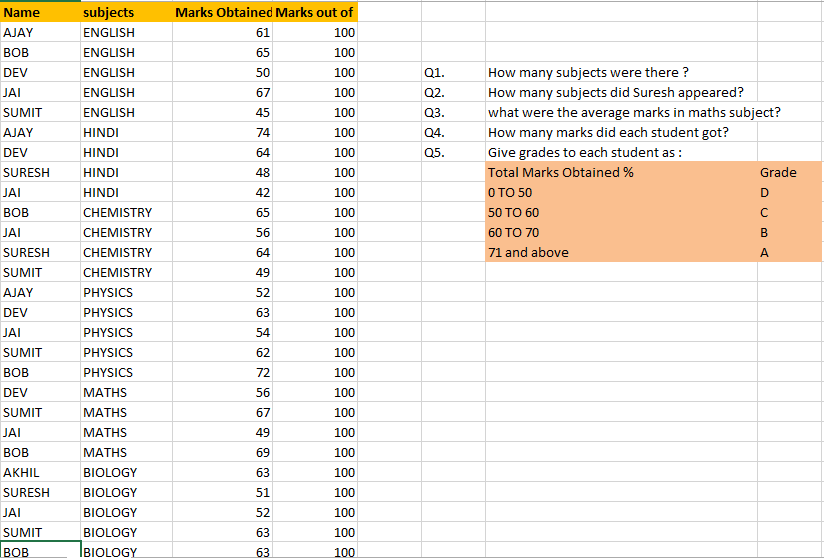Count Formulas

Accounts Tax
Excel

In this case,we have to use

=SUMPRODUCT(1/COUNTIF(SELECT RANGE,CRITERIA))

Note:-

HERE,

Range and Criteria are same,hence Formula actually is

=SUMPRODUCT(1/COUNTIF(SELECT RANGE,SELECT RANGE))

EXAMPLE